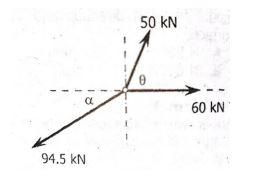# Question Three concurrent and coplanar forces in equilibrium is as shown in the figure. a. What is the value of the angle θ? b. What is the value of the angle α? c. What is the value of the horizontal component of the 94.5 KN force? 50 KN ملا 94.5 N 60 kNHGRTAY The Asker · Mechanical Engineering

Three concurrent and coplanar
forces in equilibrium is as shown
in the figure.
a. What is the value of the angle
θ?
b. What is the value of the angle
α?
c. What is the value of the
horizontal component of the
94.5 KN force?Transcribed Image Text: 50 KN ملا 94.5 N 60 kN
More
Transcribed Image Text: 50 KN ملا 94.5 N 60 kN